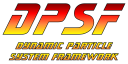#DPSFby Daniel Schroeder<PackageReference Include="DPSF" Version="2.5.0" />

# Position2D

public class Position2D
Class used to hold and update an object's 2D Position, Velocity, and Acceleration
public Vector2 Acceleration

The object's Acceleration

public Vector2 Position

The object's 2D Position

public Vector2 Velocity

The object's Position

public Vector3 AccelerationVector3 { get; set; }

Get / Set the object's 2D Acceleration using a Vector3.

NOTE: The Z-value is ignored when Setting, and is given a value of zero when Getting.

NOTE: This function is provided for convenience when using 3D Vectors in 2D coordinate systems.

para>

public Vector3 PositionVector3 { get; set; }

Get / Set the object's 2D Position using a Vector3.

NOTE: The Z-value is ignored when Setting, and is given a value of zero when Getting.

NOTE: This function is provided for convenience when using 3D Vectors in 2D coordinate systems.

public Vector3 VelocityVector3 { get; set; }

Get / Set the object's 2D Velocity using a Vector3.

NOTE: The Z-value is ignored when Setting, and is given a value of zero when Getting.

NOTE: This function is provided for convenience when using 3D Vectors in 2D coordinate systems.

para>

public Position2D()

Default Constructor

public Position2D(Position2D cPositionToCopy)

Copy Constructor

public virtual void CopyFrom(Position2D cPositionToCopy)

Copies the given Position2D object's data into this objects data

public virtual void Update(float fElapsedTimeInSeconds)

Update the Position and Velocity according to the Acceleration